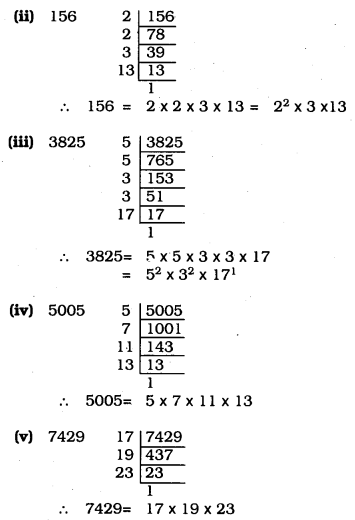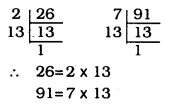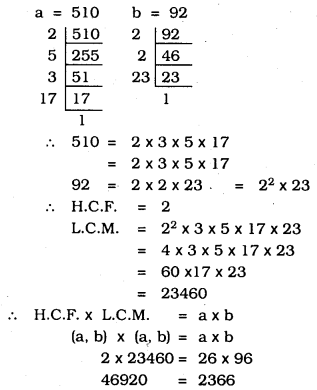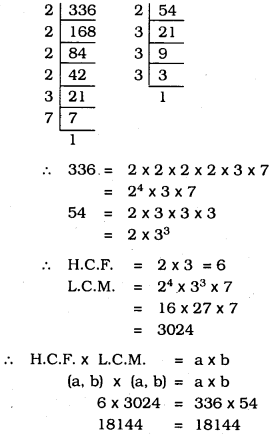# KSEEB SSLC Class 10 Maths Solutions Chapter 8 Real Numbers Ex 8.2

KSEEB SSLC Class 10 Maths Solutions Chapter 8 Real Numbers Ex 8.2 are part of KSEEB SSLC Class 10 Maths Solutions. Here we have given Karnataka SSLC Class 10 Maths Solutions Chapter 8 Real Numbers Exercise 8.2.

## Karnataka SSLC Class 10 Maths Solutions Chapter 8 Real Numbers Exercise 8.2

Question 1.
Express each number as a product of its prime factors :
(i) 140
(ii) 156
(iii) 3825
(iv) 5005
(v) 7429
Solution:Question 2.
Find the LCM and HCF of the following pairs of integers and verify that LCM × HCF = product of the two numbers.
(i) 26 and 91
(ii) 510 and 92
(iii) 336 and 54
Solution:
(i) 26 and 91
a = 26, b = 9126 = 2 × 13
91 = 7 × 13
LCM of (26, 91) = 2 × 13 × 7 = 182
HCF of (26, 91) = 13
Verification
LCM of (26, 91) × H C F (26, 91) = 182 × 13 = 2366
product of two numbers = 26 × 91 = 2366
Hence LCM × HCF = Product of two numbers.

(ii) 510 and 92
a = 510, b = 92(iii) 336 and 54
a = 336, b = 54Question 3.
Find the LCM and HCF of the following integers by applying the prime factorization method.
(i) 12, 15 and 21
(ii) 17, 23 and 29
(iii) 8, 9 and 25
Solution:
(i) 12, 15 and 21
12 = 2 × 2 × 3
15 = 3 × 5
21 = 3 × 7
∴ H.C.F. = 3
L.C.M. = 3 × 2 × 2 × 5 × 7 = 420
∴ HCF × LCM = Product of three numbers
3 × 420 = 12 × 15 × 21
1260 = 1260

ii) 17, 23 and 29
17, 23 and 29 are prime numbers
LCM of (17, 23, 29) = 17 × 23 × 29
= 391 × 29
= 11339
HCF of (17, 23, 29) = 1
(∵ 17, 23 and 29 have no common Factor)

(iii) 8, 9 and 25
8 = 2 × 2 × 2= 23
9 = 3 × 3 = 32
24 = 5 × 5 = 52
∴ H.C.F. = 1
L.C.M. = 23 × 32 × 52 = 8 × 9 × 25 = 1800
∴ HCF × LCM = Product of three numbers
1 × 1800= 8 × 9 × 25
1800 = 1800

Question 4.
Given that HCF (306, 657) = 9, find LCM (306, 657).
Solution:
H.C.F × L.C.M = a × b
(a, b) × (a, b) = a × b
9 × x = 306 × 657
x = $$\frac{306 \times 657}{9}$$
∴ x = 22338
∴ L.C.M of (306, 657) = 22338

Question 5.
Check whether 6n can end with the digit 0 for any natural number ‘n’.
Solution:
If the number ends with digit 0, then the prime factorization of the number must contain the factors of 10 as 2 × 5.
Now the prime factorization of 6n = (2 × 3)n = 2n × 3n
Since it does not contain the factors of 10 as 2 × 5, thus n cannot end with the digit 0, for any natural number n.

Question 6.
Explain why 7 × 11 × 13 + 13 and 7 × 6 × 5 × 4 × 3 × 2 × 1 + 5 are composite numbers.
Solution:
i) 7 × 11 × 13 + 13 = 13(77 + 1)
= 13 × 78
∴ It is a composite number
[It is having more than two factors]

ii) 7 × 6 × 5 × 4 × 3 × 2 × 1 + 5
=5[7 × 6 × 4 × 3 × 2 × 1 + 1]
= 5 (1008 + 1)
= 5 × 1009 = 5045
∴ It is a composite number.

Question 7.
There is a circular path around a sports field. Sonia takes 18 minutes to drive one round of the field, while Ravi takes 12 minutes for the same. Suppose they both start at the same point and at the same time and go in the same direction. After how many minutes will they meet again at the starting
Solution:
Sonia takes 18 minutes to drive one round of the field.
Ravi takes 12 minutes for the same.
Suppose they both start at the same point and at the same time.
Time to meet both again at the starting point,
For this, we have to find out HCF of 18 and 12.
18 = 2 × 3 × 3 = 2 × 32
12 = 2 × 2 × 3 = 22 × 3
∴ H.C.F. = 2 × 3 = 6
∴ After 6 minutes, they both meet at the same point.

We hope the given KSEEB SSLC Class 10 Maths Solutions Chapter 8 Real Numbers Ex 8.2 will help you. If you have any query regarding Karnataka SSLC Class 10 Maths Solutions Chapter 8 Real Numbers Exercise 8.2, drop a comment below and we will get back to you at the earliest.

error: Content is protected !!